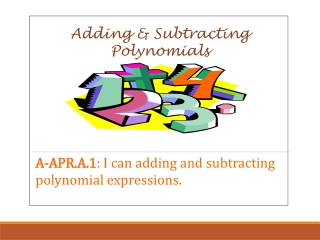DownloadDownload PresentationTélécharger la présentation- - - - - - - - - - - - - - - - - - - - - - - - - - - E N D - - - - - - - - - - - - - - - - - - - - - - - - - - -
##### Presentation Transcript

1. Adding & Subtracting Polynomials A-APR.A.1: I can adding and subtracting polynomial expressions.

2. Steps to Adding or Subtracting Polynomials • Identify like terms • Add or subtract only the coefficients of the like terms. Note: 3. Write the simplified expression in standard form

5. Adding: Polynomials • Vertically:up and down

6. How to subtract polynomials https://www.youtube.com/watch?v=5ZdxnFspyP8

7. Subtracting (Add the opposite ) • Horizontally: across

8. Subtracting (Add the opposite ) Verticallyup and down